# 机器学习算法入门之监督学习（I）TUSHARE  金融与技术学习兴趣小组0 × 1 = 0

0 × 0 = 0

0 × (-1) = 0

0 × 1/3 = 0

0 × √2 = 0

......

0 × 2 = ？

0 × (-3) = ？

0 × 1/4 = ？

0 × √3 = ？

......

① 在训练数据集中提取特征（学习），即找规律；

② 找到一个规律（模型）；

③ 根据这个规律（模型）对未知数据进行预测。

【工具】Python 3

【数据】tushare.pro

【注】本文注重的是方法的讲解，请大家灵活掌握。

01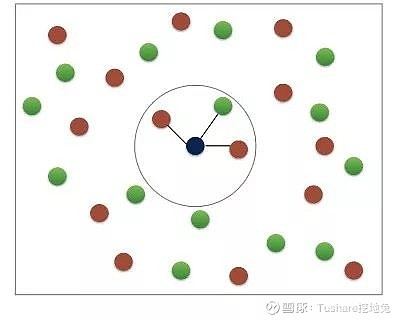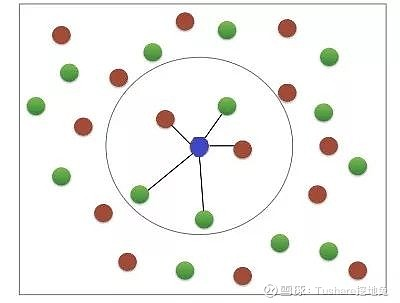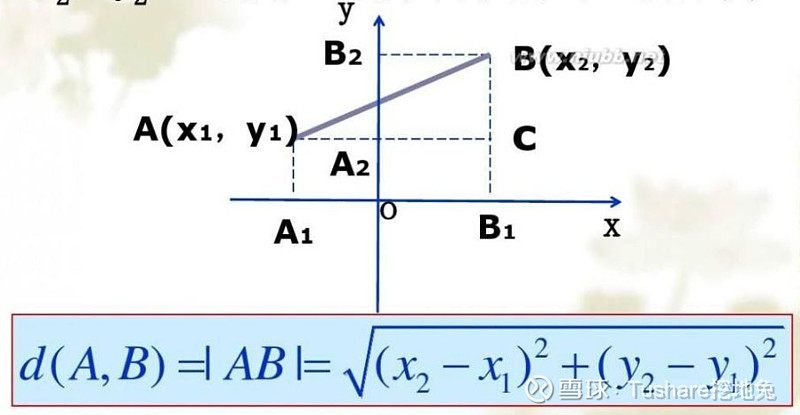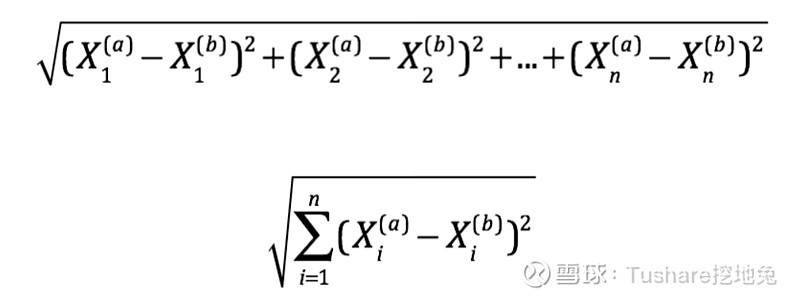import tushare as ts
import pandas as pd
import matplotlib.pyplot as plt
import seaborn as sns
pd.set_option("expand_frame_repr", False)       # 当列太多时不换行
plt.rcParams['font.sans-serif'] = ['SimHei']    # 用来正常显示中文标签
plt.rcParams['axes.unicode_minus'] = False      # 用来正常显示负号
ts.set_token('your token')
pro = ts.pro_api()
# 导入000002.SZ前复权日线行情数据，保留收盘价列
df = ts.pro_bar(ts_code='000002.SZ', adj='qfq', start_date='20190101', end_date='20190930')
df = df[['close']]
close
2019-01-02  22.97
2019-01-03  23.14
2019-01-04  23.97
2019-01-07  24.08
2019-01-08  24.03
# 计算当前、未来1-day涨跌幅
df['1d_future_close'] = df['close'].shift(-1)
df['1d_close_future_pct'] = df['1d_future_close'].pct_change(1)
df['1d_close_pct'] = df['close'].pct_change(1)
df['ma5'] = df['close'].rolling(5).mean()
df['ma5_close_pct'] = df['ma5'].pct_change(1)
df.dropna(inplace=True)
feature_names = ['当前涨跌幅方向', 'ma5当前涨跌幅方向']
df.loc[df['1d_close_future_pct'] > 0, '未来1d涨跌幅方向'] = '上涨'
df.loc[df['1d_close_future_pct'] df.loc[df['1d_close_pct'] > 0, '当前涨跌幅方向'] = 1    # 上涨记为1
df.loc[df['1d_close_pct'] df.loc[df['ma5_close_pct'] > 0, 'ma5当前涨跌幅方向'] = 1
df.loc[df['ma5_close_pct'] feature_and_target_cols = ['未来1d涨跌幅方向'] + feature_names
df = df[feature_and_target_cols]
未来1d涨跌幅方向  当前涨跌幅方向  ma5当前涨跌幅方向
2019-01-09        下跌      1.0         1.0
2019-01-10        上涨      0.0         1.0
2019-01-11        下跌      1.0         1.0
2019-01-14        上涨      0.0         0.0
2019-01-15        上涨      1.0         1.0
from sklearn.neighbors import KNeighborsClassifier
from sklearn.model_selection import train_test_split
# 创建特征 X 和标签 y
y = df['未来1d涨跌幅方向'].values
X = df.drop('未来1d涨跌幅方向', axis=1).values
# 划分训练集和测试集
X_train, X_test, y_train, y_test = train_test_split(X, y, test_size=0.2, random_state=42, stratify=y)
# 创建一个k为6的k-NN分类器
knn = KNeighborsClassifier(n_neighbors=6)
# 放入训练集数据进行学习
knn.fit(X_train, y_train)
# 在测试集数据上进行预测
new_prediction = knn.predict(X_test)
print("Prediction: {}".format(new_prediction))
# 测算模型的表现：预测对的个数 / 总个数
print(knn.score(X_test, y_test))
Prediction: ['上涨' '下跌' '上涨' '上涨' '上涨' '上涨' '上涨' '上涨' '下跌' '上涨' '上涨' '上涨' '上涨' '上涨'
'上涨' '上涨' '上涨' '上涨' '上涨' '上涨' '上涨' '上涨' '上涨' '上涨' '下跌' '下跌' '下跌' '上涨'
'上涨' '上涨' '上涨' '上涨' '下跌' '上涨' '下跌' '上涨']0.5555555555555556

import numpy as np
# 创建用于储存训练和测试集预测准确度的数组
neighbors = np.arange(1, 15)
train_accuracy = np.empty(len(neighbors))
test_accuracy = np.empty(len(neighbors))
# 循环输入不同的 k值
for i, k in enumerate(neighbors):
# 构建knn分类器
knn = KNeighborsClassifier(n_neighbors=k)
# 用训练集数据学习
knn.fit(X_train, y_train)
# 计算在训练集数据上的准确度
train_accuracy[i] = knn.score(X_train, y_train)
# 计算在测试集数据上的准确度
test_accuracy[i] = knn.score(X_test, y_test)
print(train_accuracy)
print(test_accuracy)
# 画图
plt.title('k-NN: Varying Number of Neighbors')
plt.plot(neighbors, test_accuracy, label='测试集预测准确度')
plt.plot(neighbors, train_accuracy, label='训练集预测准确度')
plt.legend()
plt.xlabel('k的取值')
plt.ylabel('准确度')
plt.show()
[0.46099291 0.4751773  0.53900709 0.4964539  0.4964539  0.4964539
0.53900709 0.53900709 0.53900709 0.53900709 0.53900709 0.53900709
0.53900709 0.53900709]
[0.36111111 0.47222222 0.63888889 0.55555556 0.55555556 0.55555556
0.63888889 0.63888889 0.63888889 0.63888889 0.63888889 0.63888889
0.63888889 0.63888889]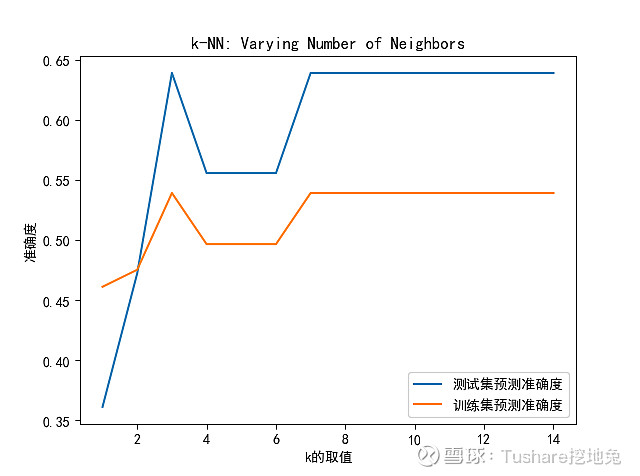02

# 导入沪深300指数、平安银行的日线涨跌幅数据
hs300 = pro.index_daily(ts_code='399300.SZ', start_date='20190101', end_date='20190930')[['trade_date', 'pct_chg']]
df_000001 = ts.pro_bar(ts_code='000001.SZ', adj='qfq', start_date='20190101', end_date='20190930')[['trade_date', 'pct_chg']]
df = pd.merge(hs300, df_000001, how='left', on='trade_date', sort=True, suffixes=['_hs300', '_000001'])
df.iloc[:, 1:] = df.iloc[:, 1:] / 100
print(df.info())
pct_chg_hs300  pct_chg_000001
2019-01-02      -0.013658         -0.0205
2019-01-03      -0.001580          0.0099
2019-01-04       0.023958          0.0512
2019-01-07       0.006070         -0.0010
2019-01-08      -0.002161         -0.0083

DatetimeIndex: 183 entries, 2019-01-02 to 2019-09-30
Data columns (total 2 columns):
pct_chg_hs300     183 non-null float64
pct_chg_000001    183 non-null float64
dtypes: float64(2)
memory usage: 4.3 KB
None
# 画图：查看相关性
plt.figure()
sns.heatmap(df.corr(), annot=True, square=True, cmap='RdYlGn')
plt.show()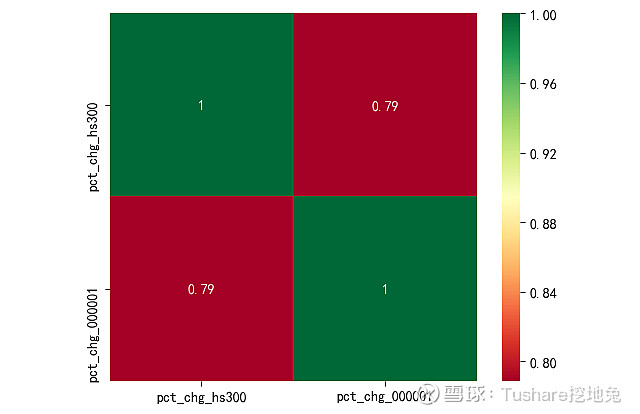sklearn.linear_model模块调用LinearRegression类做线性回归。

from sklearn.linear_model import LinearRegression
from sklearn.model_selection import train_test_split
import numpy as np
# 创建特征和标签
y = df['pct_chg_hs300'].values
X = df['pct_chg_000001'].values
print("转换前y的维度: {}".format(y.shape))
print("转换前X的维度: {}".format(X.shape))
# 转换成 n × 1维数组
y = y.reshape(-1, 1)
X = X.reshape(-1, 1)
print("转换后y的维度: {}".format(y.shape))
print("转换后X的维度: {}".format(X.shape))
# 创建训练集和测试集
X_train, X_test, y_train, y_test = train_test_split(X, y, test_size=0.3, random_state=42)
# 创建线性回归模型
reg_all = LinearRegression()
# 用训练集数据学习
reg_all.fit(X_train, y_train)
# 在测试集上进行预测
y_pred = reg_all.predict(X_test)
# 计算评价指标R^2：
print("R^2: {}".format(reg_all.score(X_test, y_test)))
pct_chg_hs300  pct_chg_000001
2019-01-02      -0.013658         -0.0205
2019-01-03      -0.001580          0.0099
2019-01-04       0.023958          0.0512
2019-01-07       0.006070         -0.0010
2019-01-08      -0.002161         -0.0083转换前y的维度: (183,)转换前X的维度: (183,)转换后y的维度: (183, 1)转换后X的维度: (183, 1)R^2: 0.617019402603039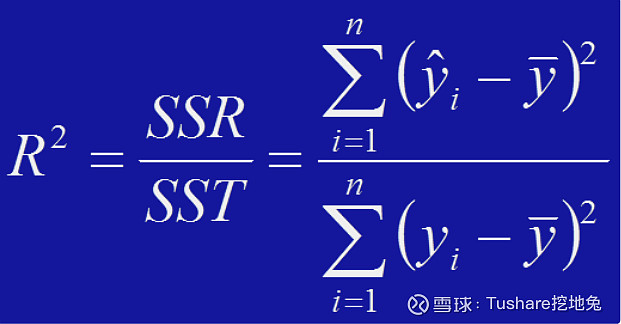K折交叉验证法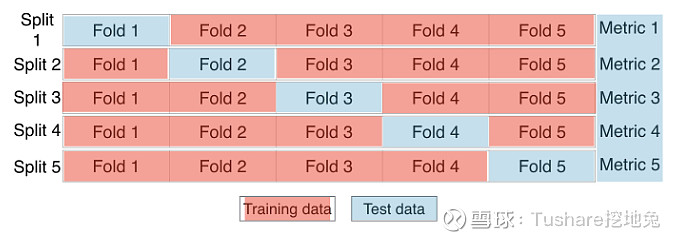from sklearn.model_selection import cross_val_score
reg = LinearRegression()
# 计算k折交叉验证得分：以k=5为例
cv_scores = cross_val_score(reg, X, y, cv=5)
print(cv_scores)
print("Average 5-Fold CV Score: {}".format(np.mean(cv_scores)))
[ 0.65150783  0.68547646  0.70675209  0.47252459 -0.19022462]
Average 5-Fold CV Score: 0.4652072684893508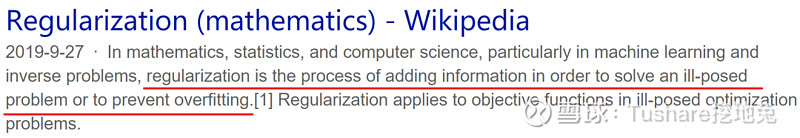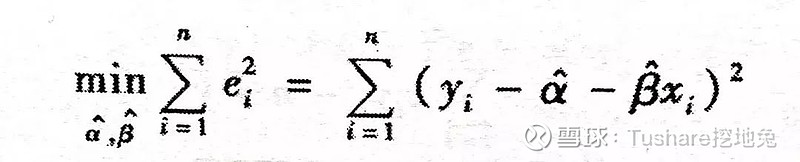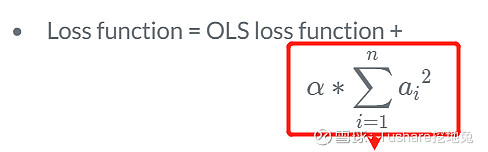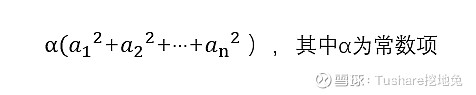# 从tushare.pro导入数据（过程省略）
print(df.info())
pct_chg_hs300  pct_chg_000001  pct_chg_000002
20190102        -0.013658         -0.0205          0.0031
20190103        -0.001580          0.0099          0.0074
20190104         0.023958          0.0512          0.0359
20190107         0.006070         -0.0010          0.0046
20190108        -0.002161         -0.0083         -0.0021

Index: 183 entries, 20190102 to 20190930
Data columns (total 3 columns):
pct_chg_hs300     183 non-null float64
pct_chg_000001    183 non-null float64
pct_chg_000002    183 non-null float64
dtypes: float64(3)
memory usage: 5.7+ KB
None
# --为了比较，计算没有惩罚项的OLS回归系数
from sklearn.linear_model import LinearRegression
reg_all = LinearRegression()
reg_all.fit(X, y)
linear_coef = reg_all.coef_
print("OLS回归系数：", linear_coef)
OLS回归系数： [[0.35175507 0.26499475]]
# --导入Ridge函数
from sklearn.linear_model import Ridge
# 创建特征和标签
y = df[['pct_chg_hs300']].values
X = df[['pct_chg_000001', 'pct_chg_000002']].values
# 创建ridge回归模型
ridge = Ridge(alpha=0.4, normalize=True)
ridge.fit(X, y)
# 计算系数
ridge_coef = ridge.coef_
print("岭回归系数：", ridge_coef)

Lasso回归

Lasso回归，也叫L1正则化，它的损失函数的数学公式如下：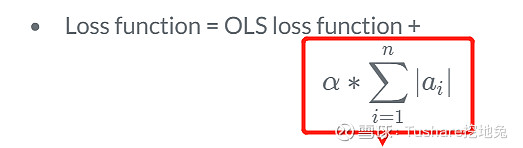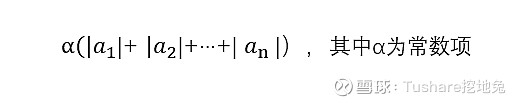Lasso回归的原理是通过使一些回归系数等于0，解决过拟合问题。同样以含有两个特征为例，切点位置落在特征2轴上，此时特征1的回归系数等于0，这样也实现了特征选择，删去了一些不重要的特征。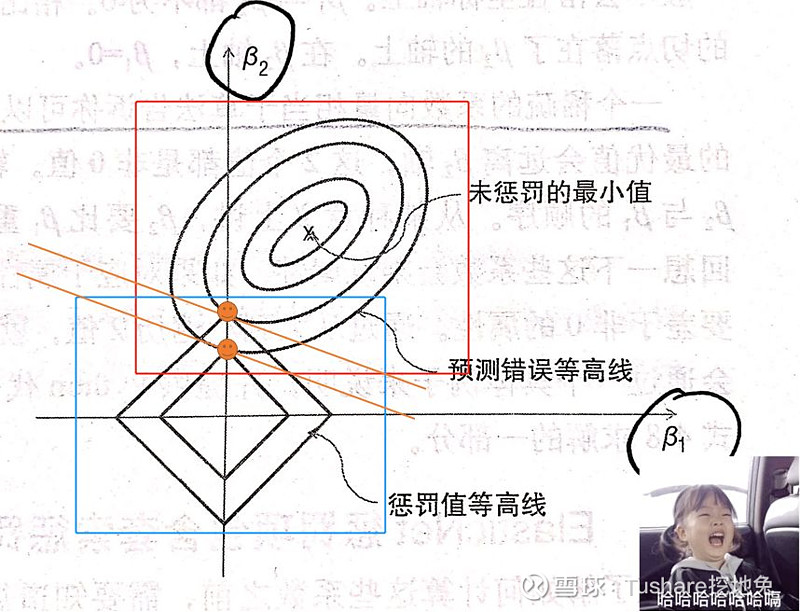# --同理，计算Lasso回归的系数from sklearn.linear_model import Lasso
lasso = Lasso(alpha=0.4, normalize=True)
lasso.fit(X, y)
lasso_coef = lasso.coef_
print("Lasso回归系数：", lasso_coef)
Lasso回归系数： [0. 0.]

03END

【参考链接】

【扩展阅读】机器学习必备技能之“统计思维2.0”机器学习必备技能之“统计思维1.0”机器学习必备技能之“数据预处理”数据科学必备基础之线性回归最简洁的Python时间序列可视化实现

 2019-10-09 11:53 雪球 转发：2 回复：1 喜欢：5

## 全部评论

AleX_7G2020-02-19 18:00